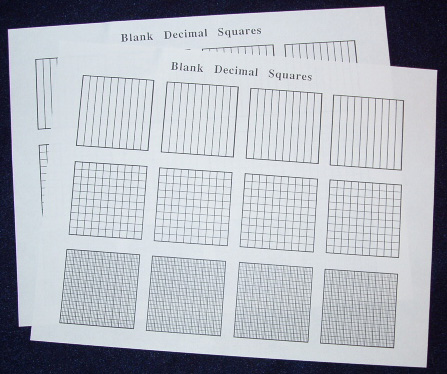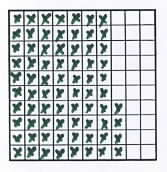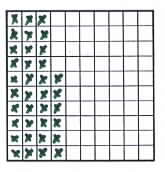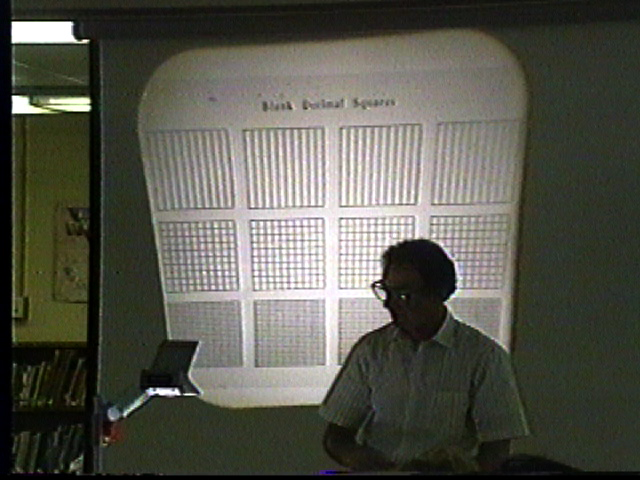Home
 Rounding . Materials: Each person will need a sheet of Blank Decimal Squares for tenths hundredths and thousandths.. We have seen that Decimal Squares provide a model for place value and they also provide an easy visual method for rounding. The following activity contains examples of rounding to the nearest tenth. Similarly shading blank thousandths squares will lead to a rule for rounding to the nearest hundredth. . Activity: Shade a blank hundredths square to represent one of the following decimals: .74 or .83. How can the shaded square be used to determine the rounded value to the nearest tenth. A discussion should produce the following rule: For a hundredths decimal if its square has a column that is less than half shaded then the decimal rounds to the given tenth (as shown on the left below); and if the square has a column that is half or more shaded then the decimal rounds to the next higher tenth as shown on the right)..74 rounds to .7 .36 rounds to .4 Another possibility for the preceding activity is to use a transparency of Blank Decimal Squares (see below) and have volunteers illustrate and explain the rounding of decimals. The squares can be used to illustrate rounding to the nearest whole number nearest tenth or nearest hundredth.Play the game ROUNDING Briefly explain this game by placing a couple of transparent square (green or yellow) on the overhead and writing in their rounded decimals in a copy of the table shown below. This game is also on page 81 of the Decimal Squares Teacher’s Guide.